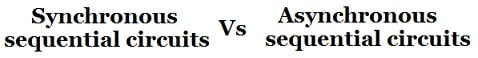# Difference between Synchronous and Asynchronous sequential circuitsThis post related to the difference between synchronous and asynchronous sequential circuits in table form. Before the differences look at the basic definitions and block diagrams of the sequential circuits and their types.

## Sequential Circuits

Sequential circuit is a combination of a combinational circuit and a memory elements connected in feedback path. The memory elements are devices capable of storing binary information within them.The binary information stored in the memory elements at any given time defines the state of the sequential circuit. The sequential circuit receives binary information from external inputs. Thus, a sequential circuit is specified by a time sequence by a time sequence of inputs, outputs and internal states.

A block diagram of sequential circuit is shown in figure below.

## Types of Sequential Circuits

There are two types of sequential circuits:

1. Synchronous sequential circuit
2. Asynchronous sequential circuit

### Synchronous sequential circuit

A sequential circuit whose output behavior depends on the input at a discrete-time is called synchronous sequential circuits. Synchronous sequential circuits that use clock pulses in the inputs of memory elements are called clocked sequential circuits.

### Asynchronous sequential circuit

The sequential circuit whose output depends on the sequence in which the input changes are called asynchronous sequential circuits.